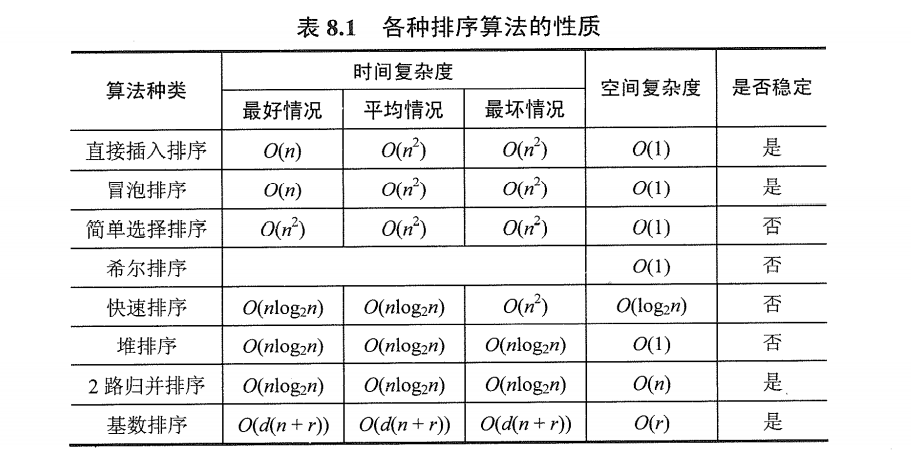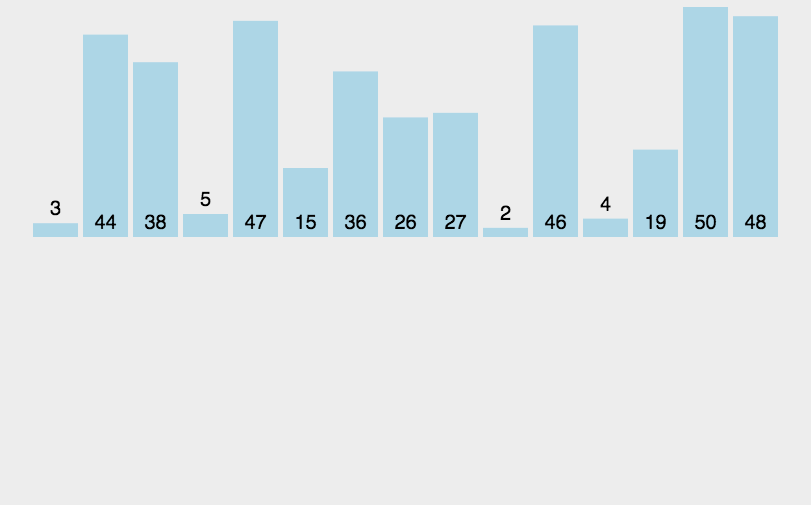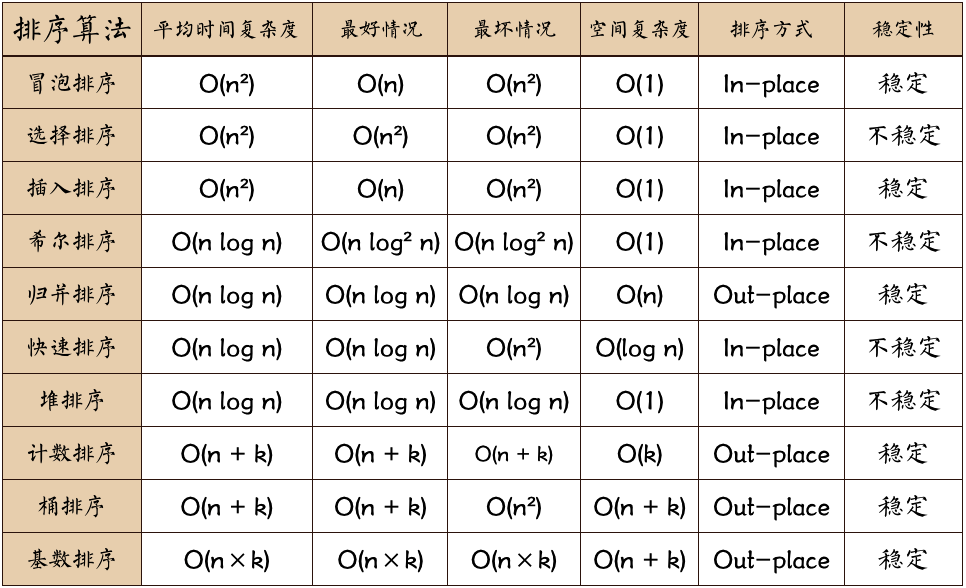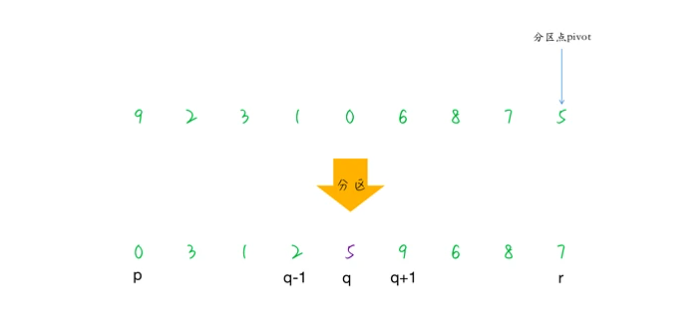+关注继续查看

# C/C++中的经典排序算法总结

## 1、冒泡排序

#include<iostream>
using namespace std;

void BulleSort(int* pData, int Count)
{
int iTemp=0;
for(int i=1;i<Count;i++)		//进行Count次排序，Count是排序的数的个数
{
for(int j=Count-1;j>=i;j--)	//对该轮待排序的数进行排序
{
if(pData[j]<pData[j-1])	//将大数放在前，小数放在后
{
iTemp=pData[j-1];
pData[j-1]=pData[j];
pData[j]=iTemp;
}
}
}
}

void main()
{
int data[]={10,7,8,3,0,5,1};	//输入待排序数组
BulleSort(data,7);				//冒泡排序法
for(int i=0;i<7;i++)
cout<<data[i]<<" ";
cout<<"\n";
}

## 2、鸡尾酒排序

#include<iostream>
using namespace std;

void CockTail(int* pData, int size)	//鸡尾酒排序，即双向冒泡法
{
int tail=size-1;
for(int i=0;i<tail;)
{
for(int j=tail;j>i;--j)			//第一轮，先将最小的数据排在前面
{
if(pData[j]<pData[j-1])
{
int temp=pData[j-1];
pData[j-1]=pData[j];
pData[j]=temp;
}
}
++i;							//原来i处的数据已排好，i加1

for(int j=i;j<tail;++j)			//第二轮，将最大的数据排在后面
{
if(pData[j]>pData[j+1])
{
int temp=pData[j+1];
pData[j+1]=pData[j];
pData[j]=temp;
}
}
tail--;							//原tail处数据也已排好，将其减1
}
}

void main()
{
int data[]={10,5,16,123,2,45,12,12,48,100,1000};
//	CockTail(data, 11);
CockTail(data, sizeof(data) / sizeof(int));	//求data数组大小的另一种方法
for(int i=0;i<11;i++)
cout<<data[i]<<" ";
cout<<"\n";
int a=sizeof(data) / sizeof(int);			//验证“求data数组大小的另一种方法”的正确性
cout<<"a="<<a<<endl;
}

## 3、选择排序

#include<iostream>
using namespace std;

void SelectSort(int* pData, int Count)
{
int iTemp;	//一个存储值
int iPos;	//一个存储下标

for(int i=0;i<Count-1;i++)
{
iTemp=pData[i];
iPos=i;
for(int j=i+1;j<Count;j++)
{
if(pData[j]<iTemp)
{
iTemp=pData[j];		//找出最小的，赋值给iTemp
iPos=j;				//并把对应的下表给iPos
}
}
pData[iPos]=pData[i];		//交换
pData[i]=iTemp;
}
}

void main()
{
int data[]={10,9,8,7,6,15,4,3};
int size=sizeof(data) / sizeof(int);
SelectSort(data,size);
for(int i=0;i<size;i++)
cout<<data[i]<<" ";
cout<<"\n";
}

## 4、直接插入排序

#include<iostream>
using namespace std;

void InsertSort(int* pData, int Count)			//直接插入排序法
{
int iTemp;
int iPos;
for(int i=1;i<Count;i++)
{
iTemp=pData[i];
iPos=i-1;
while( (iPos>=0)&&(iTemp<pData[iPos]) )
{
pData[iPos+1]=pData[iPos];
iPos--;
}
pData[iPos+1]=iTemp;
}
}

void main()
{
int data[]={100,10,2,5,41,10,23,15,2};
int size=sizeof(data)/sizeof(int);
InsertSort(data,size);
for(int i=0;i<size;i++)
cout<<data[i]<<" ";
cout<<endl;
}

## 5、归并排序

#include<iostream>
#include<malloc.h>
using namespace std;

void merge(int data[], int p, int q, int r)			//将两个有序的序列合并成一个有序的序列
{
int i,k;
int begin1,end1,begin2,end2;
int* temp=(int*)malloc((r-p+1)*sizeof(int));	//分配空间容纳临时结果，该空间大小是两个序列大小之和
begin1=p;	end1=q;
begin2=q+1;	end2=r;
k=0;
//两个序列数值大小参差不齐，要将两个有序序列的元素依次进行比较
while((begin1<=end1)&&(begin2<=end2))
{
if(data[begin1]<data[begin2])
{
temp[k]=data[begin1++];
}
else
{
temp[k]=data[begin2++];
}
k++;
}
//如果左序列有些元素比右序列的所有元素都大时，经过上一步后将左序列剩下的这些元素直接附加到temp的末尾
while(begin1<=end1)
{
temp[k++]=data[begin1++];
}
//如果右序列有些元素比左序列的所有元素都大时，经过上一步后将右序列剩下的这些元素直接附加到temp的末尾
while(begin2<=end2)
{
temp[k++]=data[begin2++];
}

for(i=0;i<(r-p+1);i++)		//将临时变量存储的结果保存在data中
{
data[p+i]=temp[i];
free(temp);
}

}

void MergeSort(int data[], unsigned int first, unsigned int last)	//实现归并排序
{
int mid=0;
if( first<last )
{
mid=(first+last)/2;				//将原数组以中间的元素为基准分为两个序列
MergeSort(data, first, mid);	//对左序列进行归并排序
MergeSort(data, mid+1, last);	//对右序列进行归并排序
merge(data, first,mid, last);	//对排序后的左右序列进行合并
}
}

void main()
{
int data[]={1,5,2,3,6,100,1,125,156,15,852};
int size=sizeof(data)/sizeof(int);
MergeSort(data, 0, size-1);
for(int i=0;i<size;i++)
cout<<data[i]<<" ";
cout<<"\n";
}

## 6、快速排序

#include<iostream>
using namespace std;

inline void swap(int v[], int j, int k)	//swap()函数：交换v[j]与v[k]的值
{
int temp;
temp=v[j];
v[j]=v[k];
v[k]=temp;
}

void qsort(int v[], int left, int right)//执行快速排序
{
int last;
if( left>=right )					//若数组包含的元素个数少于两个则直接返回
{
return;
}

swap(v, left, (left+right)/2);		//将中间的元素移到v
last=left;							//用last记录比中间值小的最右位置

for(int i=left+1;i<=right;i++)
{
if(v[i]<v[left])
{
swap(v,++last,i);
}
}
swap(v,left,last);					//恢复划分的元素
qsort(v,left,last-1);				//对左序列进行快速排序
qsort(v,last+1,right);				//对右序列进行快速排序
}

void main()
{
int data[]={10,15,2,158,23,10,25,147,189};
int size=sizeof(data)/sizeof(int);
qsort(data,0,size-1);
for(int i=0;i<size;i++)
cout<<data[i]<<" ";
cout<<"\n";
}

## 7、希尔（Shell）排序

#include<iostream>
using namespace std;

int ShellPass(int* pData, int d)		//一组增量为d的希尔插入排序
{
int temp;
int k=0;
for(int i=d+1;i<13;i++)		//13代表'size+1'
{
if(pData[i]<pData[i-d])
{
temp=pData[i];
int j=i-d;
do
{
pData[j+d]=pData[j];
j=j-d;
k++;
}
while( (j>0) && (temp<pData[j]) );
pData[j+d]=temp;
}
k++;
}
return k;
}

void ShellSort(int* pData)	//希尔排序
{
int count=0;
int ShellCount=0;
int d=12;			//一般增量设置为数组元素个数，不断除以2以取小
do
{
d=d/2;
ShellCount=ShellPass(pData,d);	//做一组增量为d的希尔插入排序
count+=ShellCount;
}
while(d>1);
cout<<"希尔排序中，关键字移动次数为:"<<count<<endl;
}

void main()
{
int data[]={10,9,8,7,6,5,4,3,2,1,-10,-1};
int size=sizeof(data)/sizeof(int);
cout<<"size="<<size<<endl;	//计算数组的大小
ShellSort(data);
for(int i=0;i<size;i++)
cout<<data[i]<<" ";
cout<<endl;
}

## 8、堆排序

#include<iostream>
using namespace std;
void heapRebuild(int arr[],int root,int size);
void heapSort(int arr[],int size);
int main()
{
const int SIZE=10;
int arr[SIZE]={10,2,13,41,23,52,7,31,65,90};
cout<<"original array arr :"<<endl;
for(int i=0;i<SIZE;i++)
cout<<i+1<<" item is :"<<arr[i]<<endl;
cout<<"after heap sorting  :"<<endl;
heapSort(arr,SIZE);
for(int i=0;i<SIZE;i++)
cout<<i+1<<" item is :"<<arr[i]<<endl;
return 0;
}
void heapRebuild(int arr[],int root,int size)
{
int child=2*root+1;
if(child<=size-1)
{
int rightChild=child+1;
if(rightChild<=size-1)
if(arr[child]<arr[rightChild])
child=rightChild;
if(arr[root]<arr[child])
{
int temp=arr[child];
arr[child]=arr[root];
arr[root]=temp;
heapRebuild(arr,child,size);
}
}
}
void heapSort(int arr[],int size)
{
for(int i=size-1;i>=0;i--)
{
heapRebuild(arr,i,size);
}
int last=size-1;
for(int i=1;i<=size;i++,last--)
{
int temp=arr;
arr=arr[last];
arr[last]=temp;
heapRebuild(arr,0,last);

}

}

## 9、排序算法总结

（1）、若从冒泡排序、鸡尾酒排序、选择排序和直接插入排序这四种算法中选择，当需要的是简单的排序法时，个人认为选择排序是最好的。

（2）、归并排序和快速排序的基本原理都是基于分治法的。其区别在于：归并排序先将待排序的序列分为两部分，再对这两部分分别进行排序，这两部分排序之后再将其进行合并；而快速排序则是先选择中间值，然后把比它小的放在左边，大的放在右边，对两边分别通过递归调用快速排序，最后两种子序列是已经排序好的，直接合并就可以了。

（3）、希尔排序实际是一种复杂的插入排序，希尔排序的时间性能优于直接插入排序，但是当文件初态基本有序时直接插入排序所需要的比较和移动次数均较少。

（4）、快速排序法是最优秀的，希尔排序是最经典的一个，所以高级程序员可以优先掌握这两种排序算法。0 0【数据结构与算法】之十大经典排序算法（下）
【数据结构与算法】之十大经典排序算法（下）
0 0【数据结构与算法】之十大经典排序算法（上）
【数据结构与算法】之十大经典排序算法（上）
0 00 00 00 0C#实现所有经典排序算法
1888 0C#实现经典排序算法
875 0504424

C++课程-对象模型

GPON Class C++ SFP OLT Transce